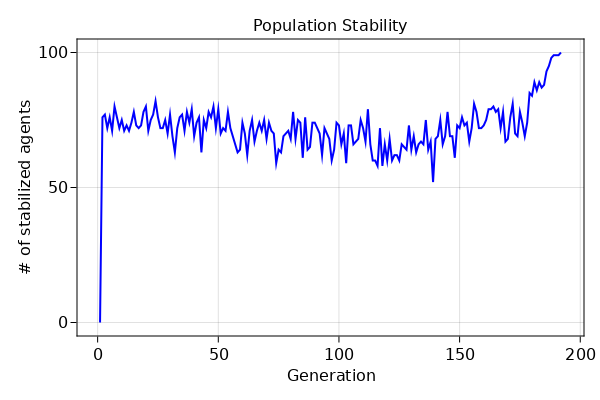This is a simple model of how an opinion spreads through a community. Each individual has a number of opinions as a list of integers. They can change their opinion by changing the numbers in the list.

Agents can change their opinion at each step. They choose one of their neighbors randomly, and adopt one of the neighbor's opinion. They are more likely to adopt their neighbors opinion if the share more opinions with each other.

using Agents
using InteractiveDynamics # plotting agents
using CairoMakie # for static plotting

## Building the model

### 1. Model creation

mutable struct Citizen <: AbstractAgent
id::Int
pos::Dims{2}
stabilized::Bool
opinion::Array{Int,1}
prev_opinion::Array{Int,1}
end

function create_model(; dims = (10, 10), nopinions = 3, levels_per_opinion = 4)
space = GridSpace(dims)
properties = Dict(:nopinions => nopinions)
model = AgentBasedModel(
Citizen,
space,
scheduler = Schedulers.randomly,
properties = properties,
)
for pos in positions(model)
pos,
model,
false,
rand(model.rng, 1:levels_per_opinion, nopinions),
rand(model.rng, 1:levels_per_opinion, nopinions),
)
end
return model
end
create_model (generic function with 1 method)

### 2. Stepping functions

function adopt!(agent, model)
neighbor = rand(model.rng, collect(nearby_ids(agent, model)))
matches = model[neighbor].opinion .== agent.opinion
nmatches = count(matches)
if nmatches < model.nopinions && rand(model.rng) < nmatches / model.nopinions
switchId = rand(model.rng, findall(x -> x == false, matches))
agent.opinion[switchId] = model[neighbor].opinion[switchId]
end
end

function update_prev_opinion!(agent, model)
for i in 1:(model.nopinions)
agent.prev_opinion[i] = agent.opinion[i]
end
end

function is_stabilized!(agent, model)
if agent.prev_opinion == agent.opinion
agent.stabilized = true
else
agent.stabilized = false
end
end

function agent_step!(agent, model)
update_prev_opinion!(agent, model)
is_stabilized!(agent, model)
end
agent_step! (generic function with 1 method)

## Running the model

First, we create a stopping condition, which runs the model until all agents stabilize.

rununtil(model, s) = count(a -> a.stabilized, allagents(model)) == length(positions(model))
rununtil (generic function with 1 method)

Then we create our model, run it and collect some information

model = create_model(nopinions = 3, levels_per_opinion = 4)

agentdata, _ = run!(model, agent_step!, dummystep, rununtil, adata = [(:stabilized, count)])
(192×2 DataFrame
Row │ step   count_stabilized
│ Int64  Int64
─────┼─────────────────────────
1 │     0                 0
2 │     1                76
3 │     2                77
4 │     3                72
5 │     4                76
6 │     5                71
7 │     6                80
8 │     7                76
⋮  │   ⋮           ⋮
186 │   185                93
187 │   186                95
188 │   187                98
189 │   188                99
190 │   189                99
191 │   190                99
192 │   191               100
177 rows omitted, 0×0 DataFrame)

## Plotting

The plot shows the number of stable agents, that is, number of agents whose opinions don't change from one step to the next. Note that the number of stable agents can fluctuate before the final convergence.

f = Figure(resolution = (600, 400))
ax =
f[1, 1] = Axis(
f,
xlabel = "Generation",
ylabel = "# of stabilized agents",
title = "Population Stability",
)
lines!(ax, 1:size(agentdata, 1), agentdata.count_stabilized, linewidth = 2, color = :blue)
f### Animation

Here is an animation that shows change of agent opinions over time. The first three opinions of an agent determines its color in RGB.

levels_per_opinion = 3
ac(agent) = CairoMakie.RGB((agent.opinion[1:3] ./ levels_per_opinion)...)
model = create_model(nopinions = 3, levels_per_opinion = levels_per_opinion)

abm_video(
"opinion.mp4",
model,
agent_step!;
ac = ac,
am = '■',
as = 20,
framerate = 20,
frames = 265,
)